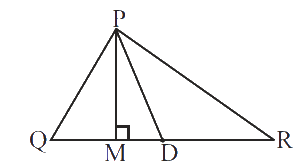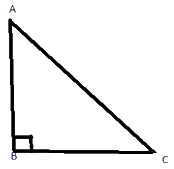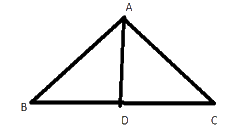# NCERT Class 7 Maths The Triangle and Its Properties

The chapter 6 begins with an introduction to triangles and its properties by explaining the elements of the triangle such as three vertices, three sides and three angles.Then the concept of medians and altitudes of a triangle are discussed in detail.Exterior angle of a triangle and its property is dealt in the next section.Similarly, the angle sum property of a triangle is described in the following section.After this, the two special triangles namely equilateral and isosceles are explained in detail.The sum of the lengths of two sides of a triangle is the next topic of discussion. The very important and interesting Right-Angled Triangle and Pythagoras Theorem is explained in the last section.

## Chapter 6 Ex.6.1 Question 1

In $$∆ PQR,$$ $$D$$ is the mid-point of is $$\overline {QR} \,.$$

$$\overline{PM}$$ _________________.

$$\overline{PD}$$is _________________.

Is $$QM ≠ MR?$$### Solution

What is known:

$$PQR$$ is a triangle, $$D$$ is the mid-point of $$QR$$.

What is unknown:

What is and . Is $$QM ≠ MR?$$

Reasoning:

Let’s visually model this problem .There are three operations that done in sequence. First, check $$PM$$ is perpendicular on $$QR$$ or not,then check $$PD$$ divides $$QR$$ in equal parts or not and then find the mid-point of $$QR$$.

According to this model, the result $$PM$$ is perpendicular on $$QR$$ then $$PD$$ divides $$QR$$ in equal parts and $$D$$ is the mid-point of $$QR$$.

Steps:

Given, $$PM$$ is perpendicular on $$QR$$. Therefore, $$PM$$ is altitude.

Also, $$D$$ is the mid-point of $$QR$$

$$QD = DR$$

$$PD$$ is median

No, $$QM ≠ MR$$ , because $$D$$ is the mid-point of $$QR$$.

## Chapter 6 Ex.6.1 Question 2

Draw rough sketches for the following:

(i) In $$∆ABC$$, $$BE$$  is a median.

(ii) In $$∆PQR$$, $$PQ$$ and $$PR$$ are altitudes of the triangle.

(iii) In $$∆XYZ$$, $$YL$$ is an altitude in the exterior of the triangle.

### Solution

What is known?

Statement.

What is unknown?

Rough sketch for given statement.

Reasoning:

Read the statement carefully and draw a rough figure according to the statement.

Steps:

Here $$BE$$ is a median in triangle$$ABC$$ therefore $$AE = EC.$$Here $$PQ$$ and $$PR$$ are the altitudes of triangle $$PQR$$ and $$PQ ┴ PR$$.$$YL$$ is an altitude in the exterior of triangle $$XYZ$$## Chapter 6 Ex.6.1 Question 3

Verify by drawing a diagram if the median and altitude of an isosceles triangle can be same.

### Solution

What is known?

An isosceles triangle.

What is unknown?

If the median and the altitude of an isosceles triangle can be same.

Reasoning:

First, draw an isosceles triangle and draw a line which divides them into two equal parts. The two sides of this triangle are equal because it is an isosceles triangle and the line drawn is perpendicular to the triangle also it divides base into two equal parts.

Steps:

Draw a triangle $$ABC$$ and then draw a line segment $$AD$$ perpendicular to $$BC$$. $$AD$$ is an Altitude of the triangle. It can be observed that length of $$BD$$ and $$DC$$ is also same. Therefore, $$AD$$ is also a median of this Triangle.The Triangle and its Properties | NCERT Solutions
Instant doubt clearing with Cuemath Advanced Math Program